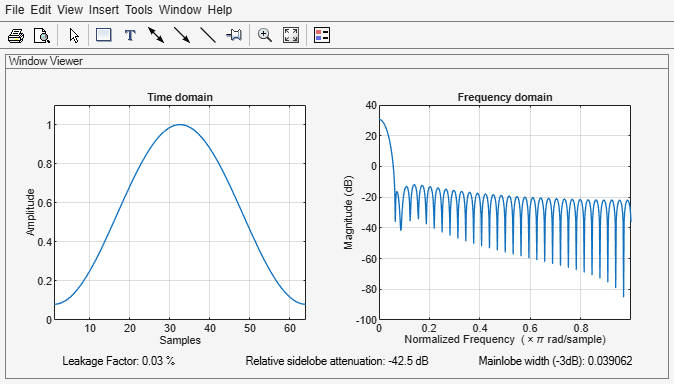# hamming

Hamming window

## Syntax

w = hamming(L)
w = hamming(L,sflag)

## Description

example

w = hamming(L) returns an L-point symmetric Hamming window.

example

w = hamming(L,sflag) returns a Hamming window using the window sampling specified by sflag.

## Examples

collapse all

Create a 64-point Hamming window. Display the result using wvtool.

L = 64; wvtool(hamming(L))Design two Hamming windows:

• The first window has N = 64 and is symmetric.

• The second window has N = 63 and is periodic.

Display the two windows.

Hs = hamming(64,'symmetric'); Hp = hamming(63,'periodic'); wvt = wvtool(Hs,Hp); legend(wvt.CurrentAxes,'Symmetric','Periodic')## Input Arguments

collapse all

Window length, specified as a positive integer.

Data Types: single | double

Window sampling method, specified as:

• 'symmetric' — Use this option when using windows for filter design.

• 'periodic' — This option is useful for spectral analysis because it enables a windowed signal to have the perfect periodic extension implicit in the discrete Fourier transform. When 'periodic' is specified, the function computes a window of length L + 1 and returns the first L points.

## Output Arguments

collapse all

Hamming window, returned as a column vector.

## Algorithms

The following equation generates the coefficients of a Hamming window:

$w\left(n\right)=\begin{array}{cc}0.54-0.46\mathrm{cos}\left(2\pi \frac{n}{N}\right),& 0\le n\le N\end{array}.$

The window length L = N + 1.

 Oppenheim, Alan V., Ronald W. Schafer, and John R. Buck. Discrete-Time Signal Processing. Upper Saddle River, NJ: Prentice Hall, 1999.